# RF Tutorial Lesson 6: Examining Coupled Transmission Lines

 Tutorial Project: Examining Coupled Transmission Lines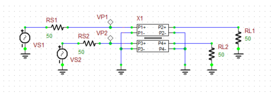Objective: In this project, you will be exposed to the concept of coupled transmission lines and will examine their properties. Concepts/Features: Coupled Transmission Lines Even Mode Odd Mode System Characteristic Impedance AC Frequency Sweep Minimum Version Required: All versions

## What You Will Learn

In this tutorial you will learn about RF.Spice's Generic Coupled T-Lines model and how to use them in your circuits.

## Introducing the Generic Coupled T-Lines Device

RF.Spice's Generic Couple T-Lines can be accessed from Menu > Parts > Transmission Lines > Generic T-Lines > Generic Coupled T-Lines. It is a four-port device that models two parallel transmission line segments with equal lengths. Coupled lines have several applications in RF circuit design including directional couplers, hybrids and filters. Normally you would use the positive pins of the four ports and ground all the four negative pins. In RF.Spice A/D, the generic coupled T-lines device is characterized by its even and odd mode impedances, Z0e and Z0o. The system characteristic impedance of the device is defined as the geometric mean of these two impedances: Z0s = √(Z0eZ0o). Even though real physical coupled line structures have slightly different effective permittivities, the average of these two is usually taken as the effective permittivity of overall transmission line. The figure below shows the property dialog of the Generic Coupled T-Lines device: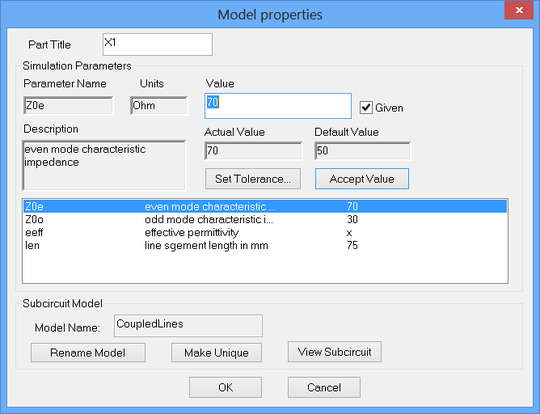The property dialog of Generic Coupled T-Lines Device.

The following is a list of parts needed for this part of the tutorial lesson:

Part Name Part Type Part Value
VS1 - VS2 AC Voltage Source 1V
X1 Generic Coupled T-Lines Z0e = 70, Z0o = 30, len = 75
RS1 - RS2 Resistor 50
RL1 - RL2 Resistor 50
VP1 - VP2 Voltage Probe Marker N/A

## Exciting the Even and Odd Modes of Coupled Lines

In the analysis of coupled transmission lines, it is customary to decompose signals as the superposition of two orthogonal modes: the even and odd modes. In this part of the tutorial lesson, you will excite each of these modes. First, you will excite the even mode. Connect the two voltage sources VS1 and VS2 through the source resistors RS1 and RS2 to the positive pins of Port 1 and Port 3 of the coupled lines device, respectively. Connect the two load resistors RL1 and RL3 to the positive pins of Port 2 and Port 4 of the coupled lines device, respectively. Place voltage probe markers VP1 and VP2 at Ports 1 and 3, respectively.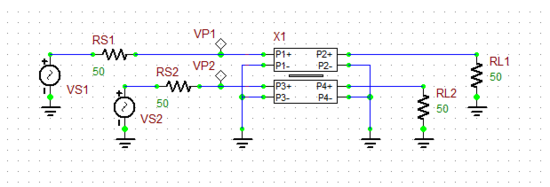Exciting the even mode of a Generic Coupled T-Lines device.

Run an AC Sweep Test of this circuit according to the table below. In the AC Sweep Test Panel, check both "Graph" and "Table" checkboxes.

 Start Frequency 500Meg 3.5G 10Meg Linear vm(vp1), vm(vp2) vr(vp1), vi(vp1), vr(vp2), vi(vp2)

After the completion of the AC sweep, you should get a voltage graph and a voltage data table similar to the ones shown below. Note from the real/imag parts table that the two input voltages are equal.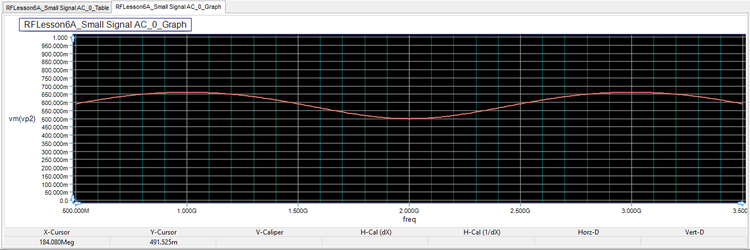Graph of the amplitude of the voltages VP1 and VP2 for the even mode excitation.Real and imaginary parts of the voltages VP1 and VP2 for the even mode excitation.

Next, you will excite the odd mode. In this case, you will connect just a single voltage source VS1 in series with the source resistor RS1 between the positive pins of Port 1 and Port 3 of the coupled lines device as shown in the opposite figure. Connect the two load resistors RL1 and RL3 to the positive pins of Port 2 and Port 4 of the coupled lines device, respectively. Place voltage probes VP1 and VP2 at Ports 1 and 3, respectively.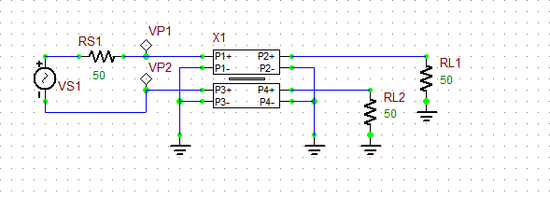Exciting the odd mode of a Generic Coupled T-Lines device.

Run an AC Sweep Test of this circuit with the same sweep settings as in the previous case. After the completion of the AC sweep, you should get a voltage graph and data table similar to the ones shown below. This time, from the real/imag parts table you can see that the two input voltages are 180° out of phase.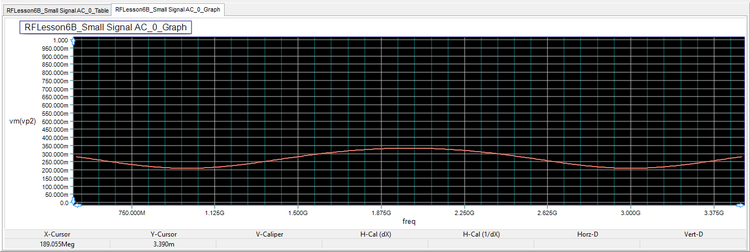Graph of the amplitude of the voltages VP1 and VP2 for the odd mode excitation.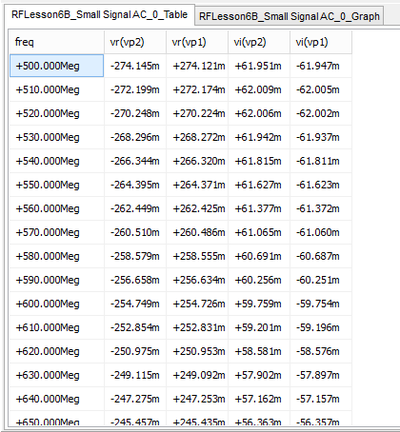Real and imaginary parts of the voltages VP1 and VP2 for the odd mode excitation.

## Coupled Mode Theory

The Generic Coupled T-Lines device is a four-port device that can easily be analyzed based on its impedance (Z) parameters. Consider the four-port being driven from Ports 1 and 3 in the even mode, with Ports 2 and 4 terminated in two identical loads ZL. The voltage at any point on either T-Line segment can be expressed as:

$V_1(z) = V_2(z) = V_e^+ \left[ e^{-j\beta (z-L)} + \Gamma_{L}^e e^{j\beta (z-L)} \right]$

where Ve+ is a constant, the coordinate z = 0 is assumed to be the location of Ports 1 and 3, and ΓLe is the load reflection coefficient for the even mode case:

$\Gamma_L^e = \frac{Z_L - Z_{0e}}{Z_L + Z_{0e}}$

The input voltages VP1 and VP2 in the even mode can now be written as:

$V_{P1} = V_1(z=0) = V_{P2} = V_2(z=0) = V_e^+ e^{j\beta L} \left[ 1 + \Gamma_{L}^e e^{-2j\beta L} \right]$

Now, let's drive the four-port from Ports 1 and 3 in the odd mode. The voltage at any point on either T-Line segment in this case can be expressed as:

$V_1(z) = -V_2(z) = V_o^+ \left[ e^{-j\beta z} + \Gamma_{L}^o e^{j\beta z} \right]$

where Vo+ is another constant, and ΓLo is the load reflection coefficient for the odd mode case:

$\Gamma_L^o = \frac{Z_L - Z_{0o}}{Z_L + Z_{0o}}$

The input voltages VP1 and VP2 in the odd mode can now be written as:

$V_{P1} = V_1(z=0) = -V_{P2} = -V_2(z=0) = V_o^+ e^{j\beta L} \left[ 1 + \Gamma_{L}^o e^{-2j\beta L} \right]$

For Z-parameter calculations, we open-circuit Ports 2 and 4 (ZL = ∞). In this case, ΓLe = ΓLo = 1, and the voltages at Ports 1 and 3 now reduce to:

$v_1^e(0) = v_2^e(0) = 2V_e^+ cos(\beta L) = i_e Z_{in}^e$

$v_1^o(0) = -v_2^o(0) = 2V_o^+ cos(\beta L) = i_o Z_{in}^o$

where ie and io are the even and odd current at Ports 1 and 3, respectively. The total currents at Ports 1 and 3 can be expressed as (ie + io) and (ie - io), respectively. Moreover, the input impedances seen at Ports 1 and 3 in the case of even and odd excitations are given by:

$Z_{in}^e = -jZ_{0e} cot( \beta L)$

$Z_{in}^o = -jZ_{0o} cot( \beta L)$

Similar equations can be derived for Ports 2 and 4, when Ports 1 and 3 are open-circuited. From all these equations, one can relate the open-circuit port voltages to the port currents and finally derive the Z matrix of the four-port as follows:

where ZS = (Z0e + Z0o)/2 and ZD = (Z0e - Z0o)/2.

The equations derived earlier for the probe voltages (VP1 and VP2) exhibit a standing wave pattern, which can clearly be observed in your node voltage graphs for both cases of even and odd mode excitations. As you already saw in Tutorial Lesson 1, the exponential term:

$e^{-j2\beta L} = e^{-j2 \frac{2\pi}{\lambda_g} L } = e^{-j2\pi f \frac{2L}{c} \sqrt{\epsilon_{eff}} }$

determines the frequency variation of the voltages, which have a periodic nature. Since εeff = 1 in this case, the period is c/(2L) = 3×108/(2×0.075) = 2GHz. You can also see from the voltage graphs that the spacing between to consecutive maxima or minima is 2GHz.

You can also verify that for the even mode excitation:

$|V_{P1}^e|_{max} = V_e^+ |\ 1+|\Gamma_L^e|\ | \\ |V_{P1}^e|_{min} = V_e^+ |\ 1-|\Gamma_L^e|\ |$

Similarly, for the odd mode excitation:

$|V_{P1}^o|_{max} = V_o^+ |\ 1+|\Gamma_L^o|\ | \\ |V_{P1}^o|_{min} = V_o^+ |\ 1-|\Gamma_L^o|\ |$

From the above equations, one can write:

$|\Gamma_L^e| = \frac{|V_{P1}^e|_{max} - |V_{P1}^e|_{min}}{|V_{P1}^e|_{max} + |V_{P1}^e|_{min}}$

$|\Gamma_L^o| = \frac{|V_{P1}^o|_{max} - |V_{P1}^o|_{min}}{|V_{P1}^o|_{max} + |V_{P1}^o|_{min}}$

Also note that in this case, ΓLe = (50-70)/(50+70) = -0.167 and ΓLo = (50-30)/(50+30) = 0.25. The table below summarizes the results obtained from the simulated data graphs:

|VP1e|max |VP1e|min |VP1o|max |VP1o|min Simulated |ΓLe| Analytical |ΓLe| Simulated |ΓLo| Analytical |ΓLo|
0.662V 0.5V 0.333V 0.209V 0.14 0.16 0.23 0.25